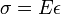# Elasticity (physics)

Elasticity, in physics and materials science, is the property of undergoing reversible deformations under applied stress. This is a generalization of the popular concept of elasticity, which generally applies only to objects which visibly stretch when pulled, and which return to their original shape when the pull is released. In physics and materials science, any deformation caused by any load which is undone when the load is removed is an elastic deformation.

Most solid materials are elastic for some range of applied load, beyond which, they undergo plastic deformation or brittle failure. Plastic deformation is a change which is not completely undone when the load is relaxed.

When the amount of deformation is directly proportional to the applied load, the material is said to be lineraly elastic. In most engineering use, considering elastic materials as linearly elastic is an acceptable approximation. When a material is linearly elastic, its elastic behavior is governed by Hooke's Law:$\sigma = E\epsilon$, where σ is the applied stress,$\epsilon$ is the strain, and E is the modulus of elasticity.

Most materials loaded in compression or tension in one direction will expand or contract in the directions perpendicular to the applied stress. The ratio of lateral strain to axial strain under a given load is Poisson's ratio, μ.Some content on this page may previously have appeared on Citizendium.